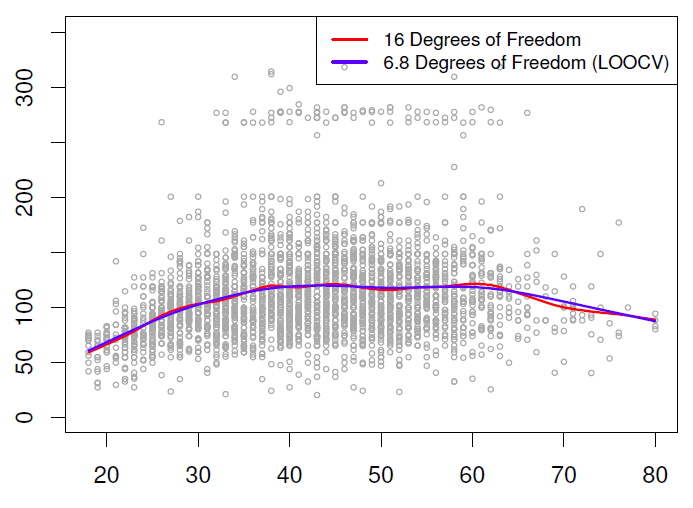# Statistics - Natural (Cubic) Spline

A natural cubic spline is a cubic spline where two extra constraints have been added at the boundaries (on each end).

The goal of this constraints is to avoid as for global cubic polynomial that the tail wag a lot. The constraints make the function extrapolate linearly beyond the boundary knots. With this constraints, the function go off linearly beyond the range of the data.

They then frees up four parameters (because of the 4 extra constraints = 2 x 2 ??). So for the same number of degrees of freedom or parameters in your model, you can put extra knots in the middle and get more flexibility.

## Degree of freedom

A natural spline with K knots has K degrees of freedom because you get back two degrees of freedom for the two constraints on each of the boundaries.

## Natural cubic spline vs …

### normal cubic spline

In the fitted function, we don't see much difference between the natural and the normal cubic spline.

• the cubic spline's a bit more wigglier at theend over here.
• the standard errors are much wider in places for the cubic spline, especially at the boundary, which is where the effect of the natural spline is taking place.
• the standard errors for the natural spline are better at the ends.

### Polynomial

Comparison of a degree-14 polynomial and a natural cubic spline, each with 15df.

In R,

ns(x, df=14)
poly(x, deg=14)


The natural cubic spline is much smoother than the very wiggly polynomial and have 14 knots. The tail of the polynomial goes crazy.

Discover MoreStatistics - Smoothing (cubic) spline

A smoothing spline is a way of fitting splines without having to worry knots. They are a little bit more challenging mathematically as others splines and approaches the problem from a completely different...Statistics - Splines

A spline is a piecewise polynomial model: Splines have the “maximum” amount of continuity over all knots function.